International
Tables for
Crystallography
Volume D
Physical properties of crystals
Edited by A. Authier

International Tables for Crystallography (2006). Vol. D, ch. 1.9, p. 228

## Section 1.9.1. Introduction

W. F. Kuhsa*

aGZG Abt. Kristallographie, Goldschmidtstrasse 1, 37077 Göttingen, Germany
Correspondence e-mail: wkuhs1@gwdg.de

### 1.9.1. Introduction

| top | pdf |

Atomic thermal motion and positional disorder is at the origin of a systematic intensity reduction of Bragg reflections as a function of scattering vector Q. The intensity reduction is given as the well known Debye–Waller factor (DWF); the DWF may be of purely thermal origin (thermal DWF or temperature factor) or it may contain contributions of static atomic disorder (static DWF). As atoms of chemically or isotopically different elements behave differently, the individual atomic contributions to the global DWF (describing the weakening of Bragg intensities) vary. Formally, one may split the global DWF into the individual atomic contributions. Crystallographic experiments usually measure the global weakening of Bragg intensities and the individual contributions have to be assessed by adjusting individual atomic parameters in a least-squares refinement.

The theory of lattice dynamics (see e.g. Willis & Pryor, 1975) shows that the atomic thermal DWF Tα is given by an exponential of the form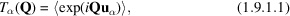where uα are the individual atomic displacement vectors and the brackets symbolize the thermodynamic (time–space) average over all contributions uα. In the harmonic (Gaussian) approximation, (1.9.1.1)reduces to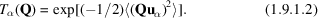The thermodynamically averaged atomic mean-square displacements (of thermal origin) are given as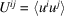, i.e. they are the thermodynamic average of the product of the displacements along the i and j coordinate directions. Thus (1.9.1.2)may be expressed with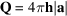in a form more familiar to the crystallographer as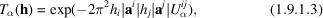whereare the covariant Miller indices,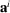are the reciprocal-cell basis vectors and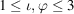. Here and in the following, tensor notation is employed; implicit summation over repeated indices is assumed unless stated otherwise. For computational convenience one often writes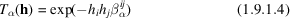with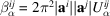(no summation). Both h and β are dimensionless tensorial quantities; h transforms as a covariant tensor of rank 1, β as a contravariant tensor of rank 2 (for details of the mathematical notion of a tensor, see Chapter 1.1).

Similar formulations are found for the static atomic DWF Sα, where the average of the atomic static displacements Δuα may also be approximated [though with weaker theoretical justification, see Kuhs (1992)] by a Gaussian distribution: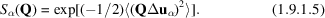As in equation (1.9.1.3), the static atomic DWF may be formulated with the mean-square disorder displacements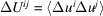as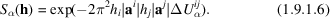It is usually difficult to separate thermal and static contributions, and it is often wise to use the sum of both and call them simply (mean-square) atomic displacements. A separation may however be achieved by a temperature-dependent study of atomic displacements. A harmonic diagonal tensor component of purely thermal origin extrapolates linearly to zero at 0 K; zero-point motion causes a deviation from this linear behaviour at low temperatures, but an extrapolation from higher temperatures (where the contribution from zero-point motion becomes negligibly small) still yields a zero intercept. Any positive intercept in such extrapolations is then due to a (temperature-independent) static contribution to the total atomic displacements. Care has to be taken in such extrapolations, as pronounced anharmonicity (frequently encountered at temperatures higher than the Debye temperature) will change the slope, thus invalidating the linear extrapolation (see e.g. Willis & Pryor, 1975). Owing to the difficulty in separating thermal and static displacements in a standard crystallographic structure analysis, a subcommittee of the IUCr Commission on Crystallographic Nomenclature has recommended the use of the term atomic displacement parameters (ADPs) for Uij and βij (Trueblood et al., 1996).

### References

Kuhs, W. F. (1992) Generalized atomic displacements in crystallographic structure analysis. Acta Cryst. A48, 80–98.
Trueblood, K. N., Bürgi, H.-B., Burzlaff, H., Dunitz, J. D., Gramaccioli, C. M., Schulz, H. H., Shmueli, U. & Abrahams, S. C. (1996). Atomic displacement parameter nomenclature. Report of a subcommittee on atomic displacement parameter nomenclature. Acta Cryst. A52, 770–781.
Willis, B. T. M. & Pryor, A. W. (1975). Thermal vibrations in crystallography. Cambridge University Press.